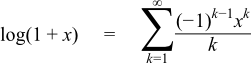#Boost C++ Libraries

...one of the most highly regarded and expertly designed C++ library projects in the world.

This is the documentation for an old version of boost. Click here for the latest Boost documentation.

### Series Evaluation

##### Synopsis
```#include <boost/math/tools/series.hpp>
```
```namespace boost{ namespace math{ namespace tools{

template <class Functor, class U, class V>
inline typename Functor::result_type sum_series(Functor& func, const U& tolerance, boost::uintmax_t& max_terms, const V& init_value);

template <class Functor, class U, class V>
inline typename Functor::result_type sum_series(Functor& func, const U& tolerance, boost::uintmax_t& max_terms);

//
// The following interfaces are now deprecated:
//
template <class Functor>
typename Functor::result_type sum_series(Functor& func, int bits);

template <class Functor>
typename Functor::result_type sum_series(Functor& func, int bits, boost::uintmax_t& max_terms);

template <class Functor, class U>
typename Functor::result_type sum_series(Functor& func, int bits, U init_value);

template <class Functor, class U>
typename Functor::result_type sum_series(Functor& func, int bits, boost::uintmax_t& max_terms, U init_value);

template <class Functor>
typename Functor::result_type kahan_sum_series(Functor& func, int bits);

template <class Functor>
typename Functor::result_type kahan_sum_series(Functor& func, int bits, boost::uintmax_t& max_terms);

}}} // namespaces
```
##### Description

These algorithms are intended for the summation of infinite series.

Each of the algorithms takes a nullary-function object as the first argument: the function object will be repeatedly invoked to pull successive terms from the series being summed.

The second argument is the precision required, summation will stop when the next term is less than tolerance times the result. The deprecated versions of sum_series take an integer number of bits here - internally they just convert this to a tolerance and forward the call.

The third argument max_terms sets an upper limit on the number of terms of the series to evaluate. In addition, on exit the function will set max_terms to the actual number of terms of the series that were evaluated: this is particularly useful for profiling the convergence properties of a new series.

The final optional argument init_value is the initial value of the sum to which the terms of the series should be added. This is useful in two situations:

• Where the first value of the series has a different formula to successive terms. In this case the first value in the series can be passed as the last argument and the logic of the function object can then be simplified to return subsequent terms.
• Where the series is being added (or subtracted) from some other value: termination of the series will likely occur much more rapidly if that other value is passed as the last argument. For example, there are several functions that can be expressed as 1 - S(z) where S(z) is an infinite series. In this case, pass -1 as the last argument and then negate the result of the summation to get the result of 1 - S(z).

The two kahan_sum_series variants of these algorithms maintain a carry term that corrects for roundoff error during summation. They are inspired by the Kahan Summation Formula that appears in What Every Computer Scientist Should Know About Floating-Point Arithmetic. However, it should be pointed out that there are very few series that require summation in this way.

##### Example

Let's suppose we want to implement log(1+x) via its infinite series,We begin by writing a small function object to return successive terms of the series:

```template <class T>
struct log1p_series
{
// we must define a result_type typedef:
typedef T result_type;

log1p_series(T x)
: k(0), m_mult(-x), m_prod(-1){}

T operator()()
{
// This is the function operator invoked by the summation
// algorithm, the first call to this operator should return
// the first term of the series, the second call the second
// term and so on.
m_prod *= m_mult;
return m_prod / ++k;
}

private:
int k;
const T m_mult;
T m_prod;
};
```

Implementing log(1+x) is now fairly trivial:

```template <class T>
T log1p(T x)
{
// We really should add some error checking on x here!
assert(std::fabs(x) < 1);

// Construct the series functor:
log1p_series<T> s(x);
// Set a limit on how many iterations we permit:
boost::uintmax_t max_iter = 1000;
// Add it up, with enough precision for full machine precision: